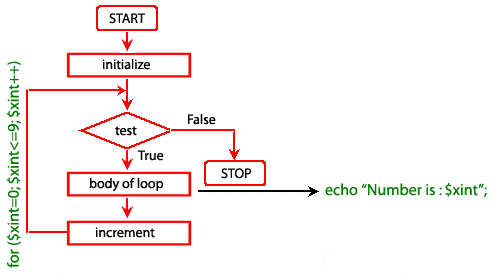# PHP for Loop

## Description

The PHP for loop allows the user to put all the loop-related statements (i.e. INITIALIZER; CONDITION; INCREMENTOR or DECREMENTOR) in one place. The structure is similar to C language.

Syntax

```for ( initialize counter ; test counter ; increment counter)
{
execute the statement;
}
```
• initialize counter : Initialize the loop counter value.
• test counter : Verify the loop counter whether the condition is true.
• increment counter : Increasing the loop counter value.
• execute the statement : Execute php statements.

Alternatively, you can write for loop statement using the following syntax also.

```for (expr1; expr2; expr3):
statement
...
endfor;
```

Pictorial representation of for loopExample:

The following example displays the numbers between 0 to 5.

``````<?php
for (\$xint=0; \$xint<=5; \$xint++)
{
echo "Number is : \$xint <br />";
}
?>``````

Output:

```Number is : 0
Number is : 1
Number is : 2
Number is : 3
Number is : 4
Number is : 5 ```

View the example in the browser

You can execute for in the following ways also.

``````<?php
for (\$xint = 1; ; \$xint++) {
if (\$i >= 5) {
break;
}
echo \$xint;
}
?>``````

``````<?php
\$xint = 1;
for (; ; )
{
if (\$xint >= 5)
{
break;
}
echo \$xint;
\$xint++;
}
?>``````

Example: Counting number of times a word present in a sentence.

``````<?php
\$text="The quick brown Fox jumps over the lazy Dog";
\$words = explode(" ", \$text); // explode function looks for " " and creates an array, where each word is an element of the array
\$now = count(\$words);
\$j = 0;
for(\$i=0; \$i<\$now; \$i++)
{
if (\$words[\$i] == "the" or \$words[\$i] == "The")
{
\$j =  \$j+1;
}
}
echo \$j;
?>``````

Output:

`2`

View the example in the browser

Previous: do-while statement
Next: foreach statement

﻿

## PHP: Tips of the Day

Members of objects or classes can be accessed using the object operator (->) and the class operator (::).

Example:

```class MyClass {
public \$a = 1;
public static \$b = 2;
const C = 3;
public function d() { return 4; }
public static function e() { return 5; }
}
\$object = new MyClass();
var_dump(\$object->a); // int(1)
var_dump(\$object::\$b); // int(2)
var_dump(\$object::C); // int(3)
var_dump(MyClass::\$b); // int(2)
var_dump(MyClass::C); // int(3)
var_dump(\$object->d()); // int(4)
var_dump(\$object::d()); // int(4)
var_dump(MyClass::e()); // int(5)
\$classname = "MyClass";
var_dump(\$classname::e()); // also works! int(5)
```

Note that after the object operator, the \$ should not be written (\$object->a instead of \$object->\$a). For the class operator, this is not the case and the \$ is necessary. For a constant defined in the class, the \$ is never used.

Also note that var_dump(MyClass::d()); is only allowed if the function d() does not reference the object:

```class MyClass {
private \$a = 1;
public function d() {
return \$this->a;
}
}
\$object = new MyClass();
var_dump(MyClass::d()); // Error!
```

This causes a 'PHP Fatal error: Uncaught Error: Using \$this when not in object context'

These operators have left associativity, which can be used for 'chaining':

```class MyClass {
private \$a = 1;

\$this->a += \$a;
return \$this;
}

public function get() {
return \$this->a;
}
}
\$object = new MyClass();
```

These operators have the highest precedence (they are not even mentioned in the manual), even higher that clone. Thus:

```class MyClass {
private \$a = 0;
\$this->a += \$a;
return \$this;
}
public function get() {
return \$this->a;
}
}
\$o1 = new MyClass();
var_dump(\$o1->get()); // int(2)
var_dump(\$o2->get()); // int(2)
```

The value of \$o1 is added to before the object is cloned!

Note that using parentheses to influence precedence did not work in PHP version 5 and older (it does in PHP 7):

```// using the class MyClass from the previous code
\$o1 = new MyClass();
\$o2 = (clone \$o1)->add(2); // Error in PHP 5 and before, fine in PHP 7
var_dump(\$o1->get()); // int(0) in PHP 7
var_dump(\$o2->get()); // int(2) in PHP 7
```